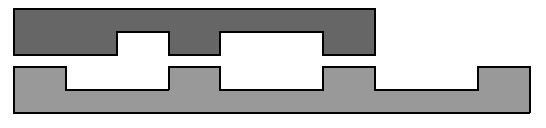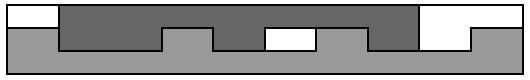# 习题3-11换抵挡转置

Kickdown

Description

A research laboratory of a world-leading automobile company has received an order to create a special transmission mechanism, which allows for incredibly efficient kickdown – an operation of switching to lower gear. After several months of research engineers found that the most efficient solution requires special gears with teeth and cavities placed non-uniformly. They calculated the optimal flanks of the gears. Now they want to perform some experiments to prove their findings.

The first phase of the experiment is done with planar toothed sections, not round-shaped gears. A section of length n consists of n units. The unit is either a cavity of height h or a tooth of height 2h . Two sections are required for the experiment: one to emulate master gear (with teeth at the bottom) and one for the driven gear (with teeth at the top).There is a long stripe of width 3h in the laboratory and its length is enough for cutting two engaged sections together. The sections are irregular but they may still be put together if shifted along each other.The stripe is made of an expensive alloy, so the engineers want to use as little of it as possible. You need to find the minimal length of the stripe which is enough for cutting both sections simultaneously.

Input

The input file contains several test cases, each of them as described below.There are two lines in the input, each contains a string to describe a section. The first line describes master section (teeth at the bottom) and the second line describes driven section (teeth at the top). Each character in a string represents one section unit – 1 for a cavity and 2 for a tooth. The sections can not be flipped or rotated.Each string is non-empty and its length does not exceed 100.

Output

For each test case, write to the output a line containing a single integer number – the minimal length of the stripe required to cut off given sections.

Sample Input

2112112112

2212112

12121212

21212121

2211221122

21212

Sample Output

10

8

15


#include<iostream>
#include<cstring>
using namespace std;
int main()
{
char s, t;
int len1, len2, len;
while(cin>>s>>t)
{
int i, j, x, y;
int len1 = strlen(s), len2 = strlen(t);
for( i=0; i<len1; i++ )
{
bool flag = true;
for( j=0; j<len2 && j+i<len1 ; j++ )
if(s[i+j]=='2'&&t[j]=='2')
{flag = false; break;}
if(flag)
break;

}
x = max(len1, len2+i);
i = j = 0;
for( i=0; i<len2; i++ )
{
bool flag = true;
for( j=0; j<len1 && j+i<len2; j++ )
if(t[i+j]=='2'&&s[j]=='2')
{flag = false; break ;}
if(flag)
break;
}
y = max(len2, len1+i);
cout<<min(x,y)<<endl;
}
return 0;
}


————————————————

©️2019 CSDN 皮肤主题: 大白 设计师: CSDN官方博客